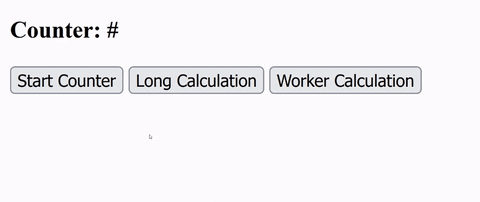## DEV Community 👩‍💻👨‍💻Youssef Zidan

Posted on • Updated on

# What are Web Workers?

## What are Web Workers?

A web worker is a JavaScript code that runs in the background and does not influence the page's performance.

As we all know, JavaScript is a single-threaded language, meaning it can only process one task at a time.

Example

``````let t = new Date();
console.log(`tastk 1 took: \${new Date() - t}ms`);
console.log(`tastk 2 took: \${new Date() - t}ms`);
console.log(`tastk 3 took: \${new Date() - t}ms`);
``````

In the above example, we see the following output in the console, In sequence just like we wrote.

``````tastk 1 took: 0ms
tastk 2 took: 0ms
tastk 3 took: 1ms
``````

Because those tasks are simple, when you open the console, you'll see that all three lines have been printed and almost no time in between.

But, What if one of the tasks took a longer time than the others?

Example

``````let t = new Date();
console.log(`tastk 1 took: \${new Date() - t}ms`);
console.log(`tastk 2 took: \${new Date() - t}ms`);
let i = 0;
while (i <= 10000000) {
i++;
}
console.log(`tastk 3 took: \${new Date() - t}ms`);
``````

In my machine, it took 2777ms to print `task 3`.

``````tastk 1 took: 0ms
tastk 2 took: 1ms
tastk 3 took: 2777ms
``````

Another Example

Copy the following code and paste it inside `index.html` file or download the GitHub Repo

index.html

``````<!DOCTYPE html>
<html lang="en">
<meta charset="UTF-8" />
<title>Web Workers</title>
<meta charset="UTF-8" />
<meta http-equiv="X-UA-Compatible" content="IE=edge" />
<meta name="viewport" content="width=device-width, initial-scale=1.0" />

<!-- counter -->
<script>
let i = 0;
let intervalId = null;
const counter = () => {
if (!intervalId) {
intervalId = setInterval(() => {
i++;
document.getElementById("counter").innerText = i;
}, 300);
} else {
clearInterval(intervalId);
i = 0;
document.getElementById("counter").innerText = i;
intervalId = null;
}
};
</script>

<!-- longCalculation -->
<script>
const longCalculation = () => {
let i = 0;
while (i <= 10000000000) {
i++;
}
};
</script>
<body>
<h3>Counter: <span id="counter"> # </span></h3>
<button onclick="counter()">Start Counter</button>
<button onclick="longCalculation()">Long Calculation</button>
</body>
</html>
``````

The first button is a simple counter that begins counting as soon as you click on it.

The other button is a piece of code that takes a long time to run.

When you click on it, you'll see that the counter along with the rest of the page is frozen until the calculation is completed.Because JavaScript is a single-threaded language, it must wait for the calculation to complete before continuing.

## Using Web Workers

This is where Web Workers come in to help.

If a process is likely to take a long time, the user is not expected to wait until it is completed. This is actually a poor user experience.

Let's create another button `Worker Calculation`.

``````<button onclick="workerCalculation()">Worker Calculation</button>
``````

Now we will add the logic of the long calculation in a separate file.

worker.js

``````let i = 0;
while (i <= 1000000000) {
i++;
}
postMessage("Worker calculation finished!");
``````

And instead of alerting the value directly, we will use the `postMessage` method.

And the logic of the `workerCalculation` function will be:

``````<script>
const workerCalculation = () => {
let worker = new Worker("worker.js");
worker.onmessage = (e) => {
};
};
</script>
``````
• Create a `worker` instance.
• Include the worker's path.
• Add an `onmessage` callback that takes an `event` as an argument

We'll use this callback to alert the `data` that comes from the `postMessage` method when the calculation is complete.The calculation will now take place in the background, and the page will not become unresponsive.

Final index.html file

``````<!DOCTYPE html>
<html lang="en">
<meta charset="UTF-8" />
<title>Web Workers</title>
<meta charset="UTF-8" />
<meta http-equiv="X-UA-Compatible" content="IE=edge" />
<meta name="viewport" content="width=device-width, initial-scale=1.0" />

<!-- counter -->
<script>
let i = 0;
let intervalId = null;
const counter = () => {
if (!intervalId) {
intervalId = setInterval(() => {
i++;
document.getElementById("counter").innerText = i;
}, 300);
} else {
clearInterval(intervalId);
i = 0;
document.getElementById("counter").innerText = i;
intervalId = null;
}
};
</script>

<!-- longCalculation -->
<script>
const longCalculation = () => {
let i = 0;
while (i <= 10000000000) {
i++;
}
};
</script>

<!-- workerCalculation -->
<script>
const workerCalculation = () => {
let worker = new Worker("worker.js");
worker.onmessage = (e) => {
};
};
</script>
<body>
<h3>Counter: <span id="counter"> # </span></h3>

<button onclick="counter()">Start Counter</button>
<button onclick="longCalculation()">Long Calculation</button>
<button onclick="workerCalculation()">Worker Calculation</button>
</body>
</html>
``````

## Browser Support

Web Workers aren't supported by all browsers.

We need to check whether the user's browser supports web workers before creating one:

``````if (typeof Worker !== "undefined") {
// Yes!
} else {
// No!
}
``````

So our worker.js file should be:

``````if (typeof Worker !== "undefined") {
let i = 0;
while (i <= 1000000000) {
i++;
}
postMessage("Worker calculation finished!");
} else {